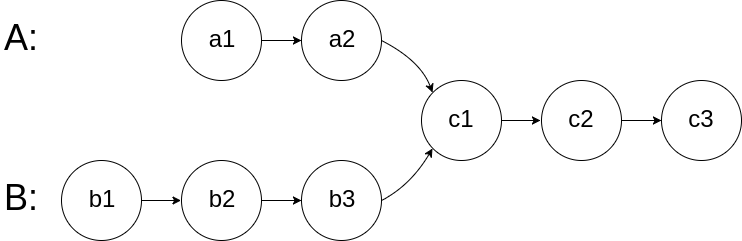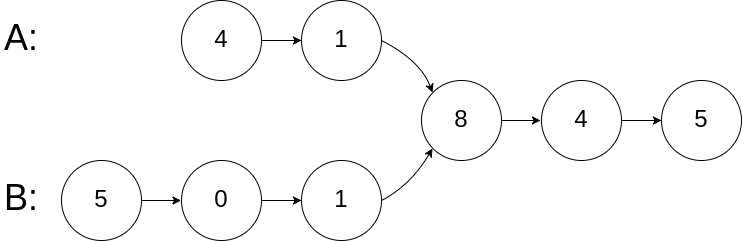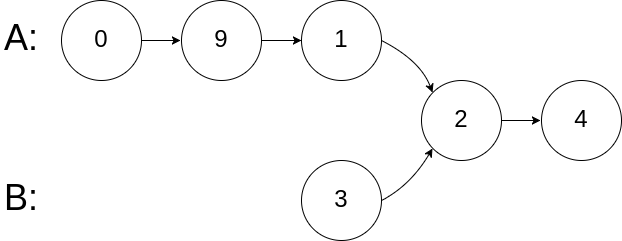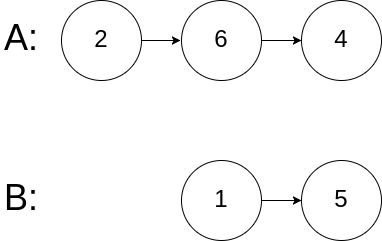0160. Intersection of Two Linked Lists

# 160. Intersection of Two Linked Lists#

## 题目 #

Write a program to find the node at which the intersection of two singly linked lists begins.

For example, the following two linked lists:begin to intersect at node c1.

Example 1:``````
Input: intersectVal = 8, listA = [4,1,8,4,5], listB = [5,0,1,8,4,5], skipA = 2, skipB = 3
Output: Reference of the node with value = 8
Input Explanation: The intersected node's value is 8 (note that this must not be 0 if the two lists intersect). From the head of A, it reads as [4,1,8,4,5]. From the head of B, it reads as [5,0,1,8,4,5]. There are 2 nodes before the intersected node in A; There are 3 nodes before the intersected node in B.

``````

Example 2:``````
Input: intersectVal = 2, listA = [0,9,1,2,4], listB = [3,2,4], skipA = 3, skipB = 1
Output: Reference of the node with value = 2
Input Explanation: The intersected node's value is 2 (note that this must not be 0 if the two lists intersect). From the head of A, it reads as [0,9,1,2,4]. From the head of B, it reads as [3,2,4]. There are 3 nodes before the intersected node in A; There are 1 node before the intersected node in B.

``````

Example 3:``````
Input: intersectVal = 0, listA = [2,6,4], listB = [1,5], skipA = 3, skipB = 2
Output: null
Input Explanation: From the head of A, it reads as [2,6,4]. From the head of B, it reads as [1,5]. Since the two lists do not intersect, intersectVal must be 0, while skipA and skipB can be arbitrary values.
Explanation: The two lists do not intersect, so return null.

``````

Notes:

• If the two linked lists have no intersection at all, return null.
• The linked lists must retain their original structure after the function returns.
• You may assume there are no cycles anywhere in the entire linked structure.
• Your code should preferably run in O(n) time and use only O(1) memory.

## 代码 #

``````
package leetcode

import "fmt"

/**
* type ListNode struct {
*     Val int
*     Next *ListNode
* }
*/
//boundary check
return nil
}

//if a & b have different len, then we will stop the loop after second iteration
for a != b {
//for the end of first iteration, we just reset the pointer to the head of another linkedlist
if a == nil {
} else {
a = a.Next
}

if b == nil {